AP State Syllabus AP Board 7th Class Maths Solutions Chapter 9 Construction of Triangles Ex 1 Textbook Questions and Answers.

## AP State Syllabus 7th Class Maths Solutions 9th Lesson Construction of Triangles Exercise 1Question 1.
Construct ∆ ABC in which AB = 5.5 cm, BC = 6.5 cm and CA = 7.5 cm.
Solution:
AB = 5.5 cm, BC = 6.5 cm, CA = 7.5 cm.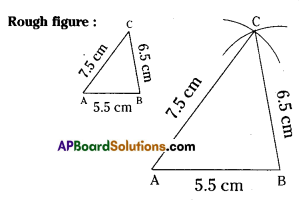Step – 1: Draw a rough sketch of the triangle and label it with the given measurements.
Step – 2: Draw a line segment AB of A length 5.5 cm.
Step – 3: With A as centre, draw an arc of radius 7.5 cm.
Step – 4: With B as centre, draw another arc of radius 6.5 cm such that it intersects first arc at C.
Step – 5: Join A, C and B, C. The required ABC is constructed.Question 2.
Construct ∆ NIB in which NI = 5.6 cm, IB = 6 cm and BN = 6 cm. What type of triangle is this?
Solution:
NI = 5.6 cm, IB = 6 cm, BN = 6 cm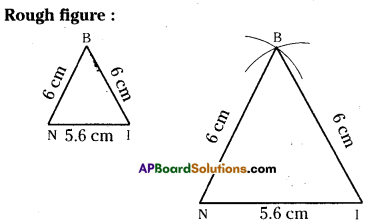Step – 1: Draw a rough sketch of the trIangle and label It with the given measurements.
Step – 2: Draw a line segment NI of length 5.6 cm. ,
Step – 3: With N as centre, draw an arc of radius 6 cm.
Step – 4: With I as centre, draw another arc of radius 6 cm such that it intersects first arc at B.
Step – 5: JoIn N, B and I, B. The required triangle ∆ NIB is constructed.
This triangle ¡s an Isosceles triangle.

Question 3.
Construct an equilateral ∆ APE with side 6.5 cm.
Solution: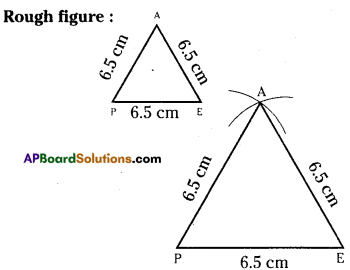AP = FE = EA : 6.5 cm.
Step – 1: Draw a rough sketch of the triangle and label it with the given measurements.
Step – 2: Draw a line segment PE of length 6.5 cm.
Step – 3: With P as centre, draw an arc of radius 6.5 cm.
Step – 4: WIth E as centre, draw another arc of radius 6.5 cm such that it intersects first arc at A.
Step – 5: Join P, A and E, A. The required ∆AFE is constructed.Question 4.
Construct a ∆ XYZ in which XY = 6 cm, YZ = 8 cm and ZX = 10 cm. Using protractor find the angle at X. What type of triangle is this?
Solution:
XY = 6cm, YZ = 8cm, ZX = 10cm.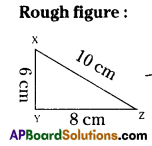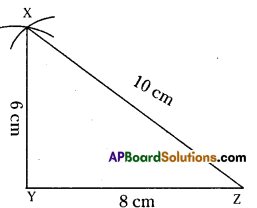Step – 1: Draw a rough sketch of the triananle and label it with the given measurements.
Step – 2: Draw a line segment YZ of length of 8 cm.
Step – 3: With Y as centre, draw an arc of radius 6 cm.
Step – 4: With Z as centre, draw another arc of radius 10 cm such that it intersects first arc at X.
Step – 5: Join Y, X and Z, X. The required XYZ is constructed ∠X = 52°
This triangle is an acute angled triangle.

Question 5.
Construct ∆ ABC in which AB =4 cm, BC = 7 cm and CA= 3 cm. Which type of triangle is this?
Solution:
AB = 4 cm, BC = 7 cm, CA = 3 cm.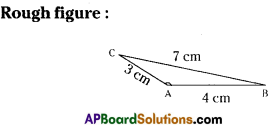Step – 1: Draw a rough sketch of the triangle and label it with the given measurements.
Step – 2: Draw a line segment AB of length 4 cm.
Step – 3: With A as centre, draw an arc of radius 3 cm.
Step – 4: With B as centre, draw another arc of radius 7 cm such that it intersects first arc at C.
Step – 5: Join C, A and C, B. The required ∆ABC is constructed.
This triangle Is an obtuse angled triangle.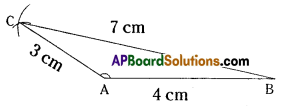Question 6.
Construct ∆ PEN with PE = 4 cm, EN =5 cm and NP =3 cm. If you draw circles instead of arcs how many points of intersection do you get? How many triangles with given measurements are possible? Is this true in case of every triangle?
Solution:PE = 4cm,EN = 5cm, NP = 3crn.
Step – 1: Draw a rough sketch of the triangle and label it with the given measurements.
Step – 2: Draw a line segment EN of length 5 cm.
Step – 3: With E as centre, draw on arc (circle) of radius 4 cm.
Step – 4: With N as centre, draw another arc (circle) of radius 3 cm such that it intersects first arc at P.
Step – 5: Join E, P and N, P. The required ∆PEN is constructed,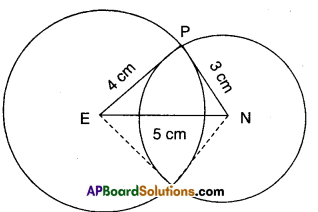if we draw circles instead of arcs, we get two points of intersection. Then, we can draw two triangles with the given measurements.
Yes, this is true in case of every triangle.